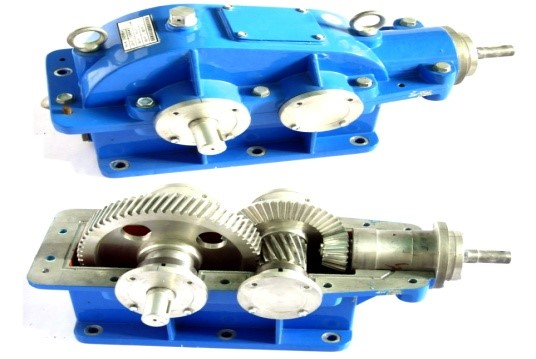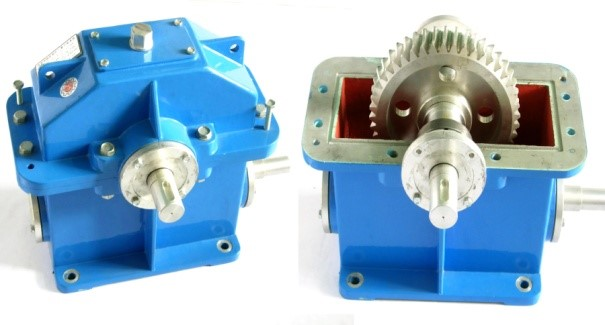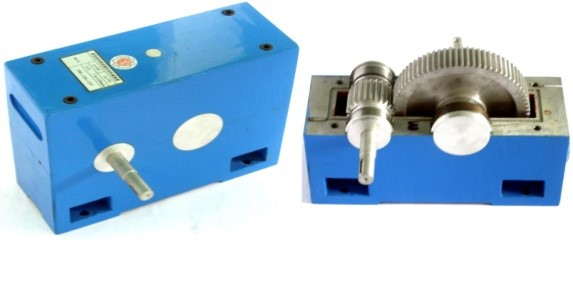2021年6月10日

#### 减速器拆装实验室,减速器拆装教学模型,拆装实验用大型减速器（全铝制）

拆装减速器制作材料除标准件外，全部用优质铝合金精加工制作而成，传动齿轮用滚齿机加工。精度高、配合正确、传动灵活，减速器外壳表面进行烤漆处理。产品精久耐用，全铝减速器我厂独家生产历史已有多年。

①、 本系列大型拆装减速器专供学生作拆装实验用，为设计和测量零件尺寸和绘制正确零部件及装配图提供了实物依据。

②、 该系列产品品种齐全、尺寸合适、重量轻、设计结构合理牢固。

③、 减速器附件类型多，开扩了学生的视野。

 序号 货物名称 技术参数及要求 数量 单位 1 展开式双级圆柱齿轮减速器 模数：m=3，齿数：Z1=16 Z2=47 Z3=27 Z4=68，压力角：20°，β：15°，传动比： i =7.398，中心高度：h =146，制作材料：箱体和轴系采用全铝合金制造，长×宽×高：535×330×294 1 台 2 单级圆柱齿轮减速器 模数：m=3，齿数：Z1=72 Z2=29，压力角：20°，β：15°，传动比： i =2.49，中心高度：h =151，制作材料：箱体和轴系采用全铝合金制造，长×宽×高：450×400×340 1 台 3 分流式双级圆柱齿轮减速器 模数：m=3，齿数:Z1=16 Z2=47 Z3=27 Z4=68，压力角：20°，β：15°，传动比：i =7.398，中心高度：h =146，制作材料：箱体和轴系采用全铝合金制造，长×宽×高：40×360×320 1 台 4 同轴式双级圆柱齿轮减速器 模数：m=3，齿数：Z1=22 Z2=26 Z3=78 Z4=82，压力角：20°，β：15°，传动比：i =11.1818，中心高度：h =159，制作材料：箱体和轴系采用全铝合金制造，长×宽×高；560×420×350 1 台 5 单级圆锥齿轮减速器 模数：m=3，齿数：Z1=20 Z2=60，压力角：20°，β：15°，传动比：i =3，中心高度：h =129，制作材料：箱体和轴系采用全铝合金制造，长×宽×高：500×350×315 1 台 6 双级圆锥圆柱齿轮减速器 模数：m=3，齿数：Z1=20 Z2=50 Z3=16 Z4=68，β：15°，传动比：i =10.625,中心高度：h =141，制作材料：箱体和轴系采用全铝合金制造，长×宽×高：550×460×360 1 台 7 蜗轮蜗杆（上置式）减速器 模数：m=4，齿数：Z1=1 Z2=45，β：15°，传动比：i =45，中心高度：h =163，制作材料：箱体和轴系采用全铝合金制造,长×宽×高：420×320×400 1 台 8 蜗轮蜗杆（下置式）减速器 模数：m=4，齿数：Z1=1 Z2=45，β：45°，传动比：i =45，中心高度：h =210，制作材料：箱体和轴系采用全铝合金制造，长×宽×高：420×320×400 1 台 9 新型单级圆柱齿轮减速器 模数：m=2，齿数：Z1=25 Z2=75；传动比：i =3；中心高度：h =120；制作材料：箱体和轴系采用全铝合金制造；长×宽×高:290×220×215 1 台▲同轴式双级圆柱齿轮减速器▲单级圆锥齿轮减速器▲双级圆锥圆柱齿轮减速器▲蜗轮蜗杆（上置式）减速器▲蜗轮蜗杆（下置式）减速器▲新型单级圆柱齿轮减速器

 空间及平面机构综合实验台
021-61482202 021-61482206